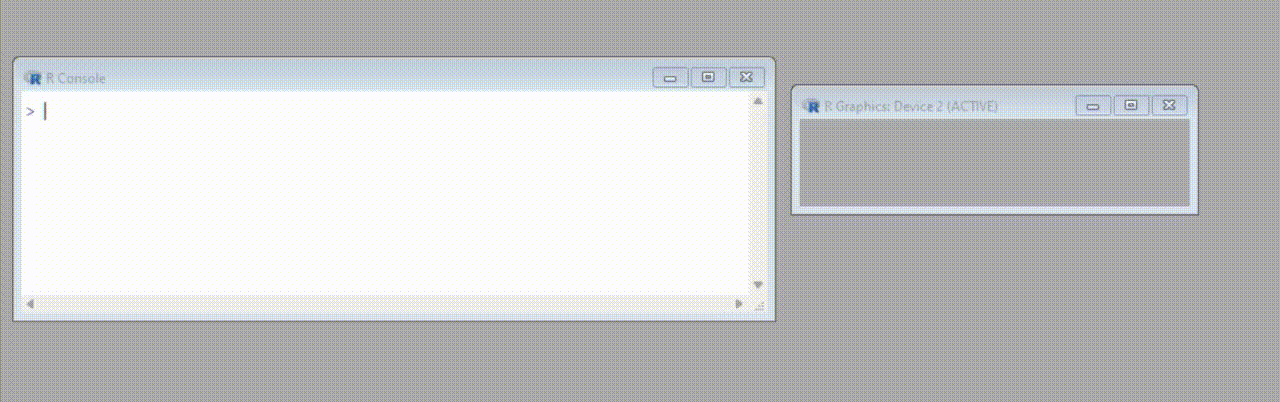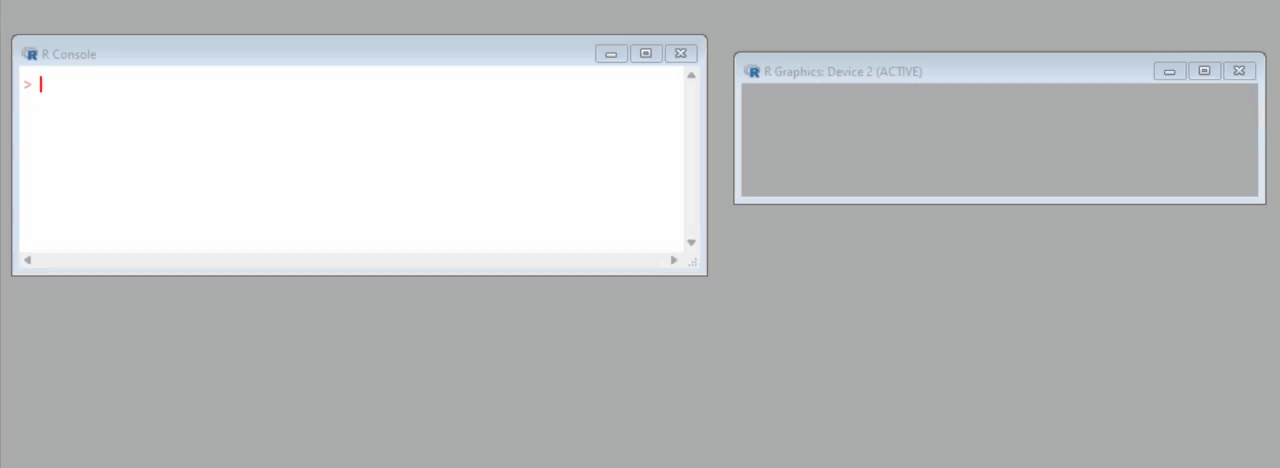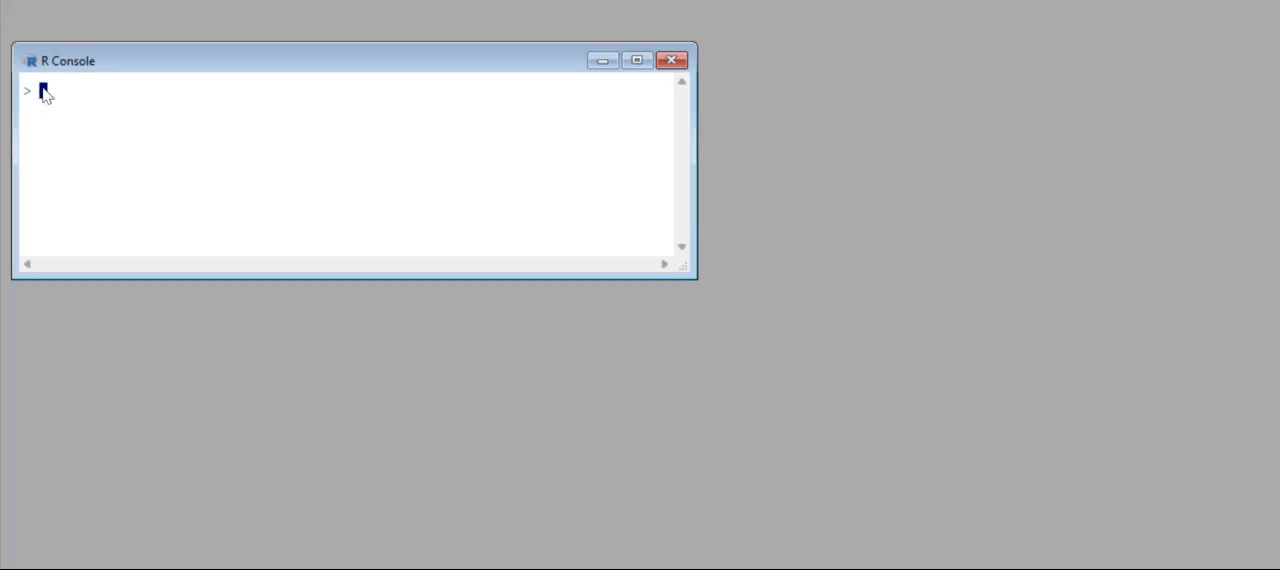GFG App
Open AppBrowser
Continue

# How to Fix in R: error in plot.new() : figure margins too large

In this article, we will discuss how to fix the “figure margins too large” error in plot.new() function of the R programming language.

The error that one may face in R is:

`Error in plot.new() : figure margins too large`

The R compiler produces this error when the plot panel of Rstudio is small for the dimensions of the plot that we are trying to create.

## When this error might occur:

Consider that want to create a plot using the plot() function in R. The syntax of this function is given below:

Syntax:

plot(start : end)

Parameters:

• start: The starting point ( 1 for (x, y) = (1, 1) etc)
• end: The ending point ( 5 for (x, y) = (5, 5) etc)

Return Type:

Draws dots in a sequence on both x and y axis

Example:

## R

 `# Draw a plot ` `plot``(1:40)`

Output:The R compiler produces the error (you can see the panel window is quite small at the right).

## How to fix this error:

There are three ways to fix this error in R:

### Method 1: Increasing the size of the panel

One way is to increase the panel size so that it can accommodate the plot across its dimensions:

## R

 `# Draw a plot ` `plot``(1:40)`

Output:### Method  2: Using par() function

The par() function in R is used to set the margins of a plot. This function has the following syntax:

Syntax:

par(mfrow)

Parameter:

mfrow:  It represents a vector with row and column values for the grid

By default a plot has the following margins:

• Top: 4.1 and Bottom: 5.1
• Left: 4.1 and Right: 2.1

We need to explicitly set the margins of the plot as:

## R

 `# Set plot margins ` `par``(mar = ``c``(1, 1, 1, 1)) ` ` `  `# Create the plot ` `plot``(1 : 40)`

Output:The plot was projected easily in the panel window because we reduced the margin to accommodate the created plot.

### Method 3: Turn off the plotting device

If neither of the previous methods was able to fix the error then you can shut down the current plotting device by the following command:

## R

 `# Turn off the device ` `dev.off``()`

Output:My Personal Notes arrow_drop_up